×#### Thank you for registering.

One of our academic counsellors will contact you within 1 working day.

Click to Chat

1800-1023-196

+91-120-4616500

CART 0

• 0

MY CART (5)

Use Coupon: CART20 and get 20% off on all online Study Material

ITEM
DETAILS
MRP
DISCOUNT
FINAL PRICE
Total Price: Rs.

There are no items in this cart.
Continue Shopping• Complete JEE Main/Advanced Course and Test Series
• OFFERED PRICE: Rs. 15,900
• View Details

```Chapter 15: Areas Related To Circles Exercise – 15.2

Question: 1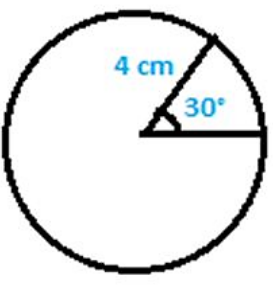Find in terms of π, the length of the arc that subtends an angle of 30 degrees, at the center of O of the circle with a radius of 4 cm.

Solution:

Given Data:

Angle subtended at the centre 'O' = 30°

Formula to be used: Length of arc = θ/360 × 2πr cm

Length of arc = 30/360 × 2π∗4 cm = 2π/3 cm

Therefore, the Length of arc the length of the arc that subtends an angle of 60 degrees is 2π/3 cm

Question: 2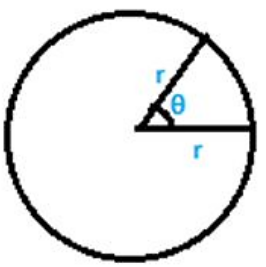Find the angle subtended at the centre of circle of radius 5 cm by an arc of length 5π/3 cm.

Solution:

Given data: Radius = 5 cm

Length of arc = 5π/3 cm

Formula to be used: Length of arc = θ/360 ∗ 2πr cm

5π/3 cm = θ/360 ∗ 2πr cm

Solving the above equation, we have:

θ = 60°

Therefore, angle subtended at the centre of circle is 60°

Question: 3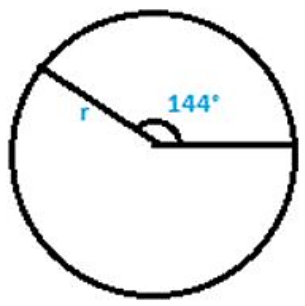An arc of length cm subtends an angle of 144° at the center of the circle.

Solution:

Given Data:

Length of arc = 25 cm

θ = Angle subtended at the centre of circle = 144°

Formula to be used:

Length of arc = θ/360 ∗ 2πr cm

θ/360 ∗ 2πr cm = 144/360 east 2πr cm = 4π/5 ∗ r cm

As given in the question, length of arc = cm,

Therefore, cm = 4π/5 ∗ r cm

Solving the above equation, we have r = 25 cm.

Therefore the radius of the circle is found to be 25 cm.

Question: 4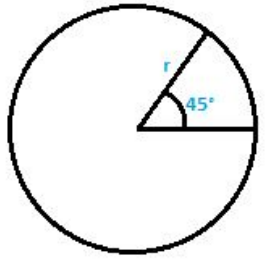An arc of length 25 cm subtends an angle of 55° at the center of a circle. Find in terms of radius of the circle.

Solution:

Given Data:

Length of arc = 25 cm

θ = Angle subtended at the centre of circle = 55°

Formula to be used:

Length of arc = θ/360 ∗ 2πr cm

= 55/36 ∗ 2πr cm

As given in the question length of arc = 25 cm, hence,

25 cm = 55/360 ∗ 2π ∗ r cm

25 = 11πr/36

Therefore, the radius of the circle is 900/11π

Question: 5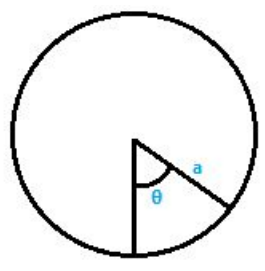Find the angle subtended at the center of the circle of radius 'a' cm by an arc of π/4 length cm.

Solution:

Given data:

Length of arc = aπ/4 cm

θ = angle subtended at the centre of circle

Formula to be used:

Length of arc = θ/360 ∗ 2πr cm

Length of arc = θ/360 ∗ 2πa cm

θ/360 ∗ 2πa cm = aπ/4 cm

Solving the above equation, we have

θ = 45°

Therefore, the angle subtended at the centre of circle is 45°

Question: 6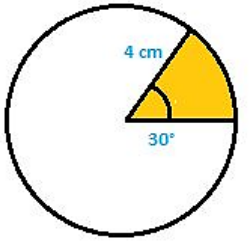A sector of the circle of radius 4 cm subtends an angle of 30°. Find the area of the sector.

Solution:

Given Data: Radius = 4 cm

Angle subtended at the centre 'O' = 30°

Formula to be used:

Area of the sector = θ/360 ∗ πr2

Area of the sector = 30/360 ∗ π42

Solving the above equation, we have: Area of the sector = 4.9 cm2

Therefore, Area of the sector is found to be 4.9 cm2

Question: 7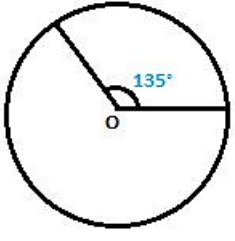A sector of a circle of radius 8 cm subtends an angle of 135. Find the area of sector.

Solution:

Given Data: Radius = 8 cm

Angle subtended at the centre 'O' = 135°

Formula to be used:

Area of the sector = θ/360 ∗ πr2

Area of the sector = 135/360 ∗ π82

= 528/7 cm2
Therefore, Area of the sector calculated is 528/7 cm2

Question: 8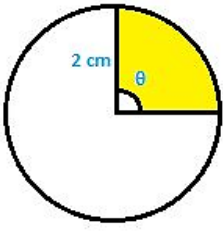The area of sector of circle of radius 2 cm is cm2. Find the angle subtended by the sector.

Solution:

Given Data: Radius = 2 cm

Angle subtended at the centre 'O'

Area of sector of circle = cm2

Formula to be used:

Area of the sector = θ/360 ∗πr2

Area of the sector = θ/360 ∗ π32

= πθ/90

As given in the question area of sector of circle = cm2

cm2 = π θ/90

Solving the above equation, we have

θ = 90°

Therefore, the angle subtended at the centre of circle is 90°

Question: 9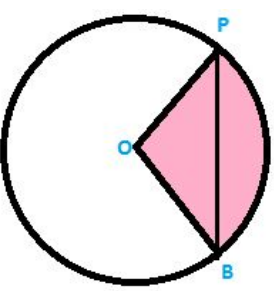PQ is a chord of circle with centre 'O' and radius 4 cm. PQ is of the length 4 cm. Find the area of sector of the circle formed by chord PQ.

Solution:

Given Data:

PQ is chord of length 4 cm. Also, PO = QO = 4 cm OPQ is an equilateral triangle.

Angle POQ = 60°

Area of sector (formed by the chord (Shaded region)) = (Area of sector)

Formula to be used:

Area of the sector = θ/360 ∗ πr2

Area of the sector = 60/360 ∗ π42 = 32π/3

Therefore, Area of the sector is 32π/3 cm2

Question: 10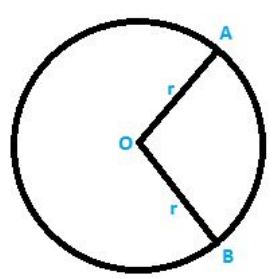In a circle of radius 35 cm, an arc subtends an angle of 72° at the centre. Find the length of arc and area of sector.

Solution:

Given Data: Radius = 35 cm

Angle subtended at the centre 'O' = 72°

Area of sector of circle =?

Formula to be used: Length of arc = θ/360 ∗ 2πr cm

Length of arc = 108/360 ∗ 2πx = 42 cm

Solving the above equation we have,

Length of arc = 44 cm

We know that,

Area of the sector = θ/360 ∗ πr2

Area of the sector = 72/360 ∗ π352

Solving the above equation, we have,

Area of the sector = (35 × 22) cm2

Therefore, Area of the sector is 770 cm2

Question: 11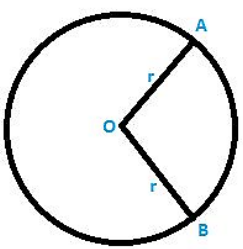The perimeter of a sector of a circle of radius 5.7 m is 27.2 m. find the area of the sector.

Solution:

Given Data:

Radius = 5.7 cm = OA = OB [from the figure shown above]

Perimeter = 27.2 m

Let the angle subtended at the centre be θ

Perimeter = θ/360 ∗ 2πr cm + OA + OB

= θ/360 ∗ 2πx 5.7 cm + 5.7 + 5.7

Solving the above equation we have,

θ = 158.8°

We know that, Area of the sector = θ/360 ∗ πr2

Area of the sector = 158.8/360 ∗ π 5. 72

Solving the above equation we have,

Area of the sector = 45.048 cm2

Therefore, Area of the sector is 45.048 cm2

Question: 12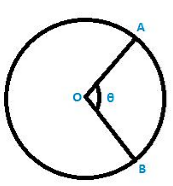The perimeter of a certain sector of a circle of radius is 5.6 m and 27.2 m. find the area of a sector.

Solution:

Given data:

Radius of the circle = 5.6 m = OA = OB

(AB arc length) + OA + OB = 27.2

Let the angle subtended at the centre be θ

We know that,

Length of arc = θ/360 ∗ 2πr cm

θ/360 ∗ 2πr cm + OA + OB = 27.2 m

θ/360 ∗ 2πr cm + 5.6 + 5.6 = 27.2 m

Solving the above equation, we have,

θ = 163.64°

We know that, Area of the sector = θ/360 ∗ πr2

Area of the sector = 163.64/360 ∗ π 5.62

On solving the above equation, we have,

Area of the sector = 44.8 cm2

Therefore,

Area of the sector is 44.8 cm2

Question: 13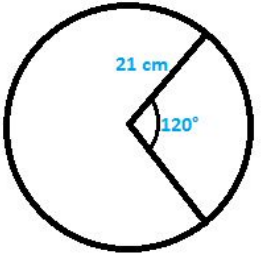A sector was cut from a circle of radius 21 cm. The angle of sector is 120°. Find the length of its arc and its area.

Solution:

Given data: Radius of circle (r) = 21 cm

θ = angle subtended at the centre of circle = 120°

Formula to be used:

Length of arc = θ/360 ∗ 2πr cm

Length of arc = 120/360 ∗ 2πx 21cm

On solving the above equation, we get, Length of arc = 44 cm

We know that, Area of the sector = θ/360 ∗ πr2

Area of the sector = 120/360 ∗ π212

Area of the sector = (22 × 21) cm2

Therefore, Area of the sector is 462 cm2

Question: 14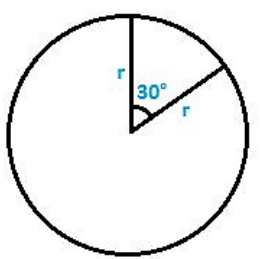The minute hand of a circle is √21 cm long. Find the area described by the minute hand on the face of clock between 7:00 a.m to 7:05 a.m.

Solution:

Given data: Radius of the minute hand (r) = √21cm

Time between 7: 00 a. m to 7: 05 a. m = 5 min

We know that, 1 hr = 60 min, minute hand completes

One revolution = 360° 60 min = 360°

θ = Angle subtended at the centre of circle = 5 × 6° = 30°

Area of the sector = θ/360 ∗ πr2

Area of the sector = 30/360 ∗ pi352

Area of the sector = 5.5 cm2

Therefore, Area of the sector is 5.5 cm2

Question: 15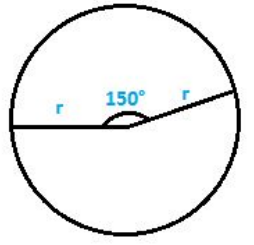The minute hand of clock is 10 cm long. Find the area of the face of the clock described by the minute hand between 8 a. m to 8:25 a.m.

Solution:

Given data: Radius of the circle = radius of the clock = length of the minute hand = 10 cm

We know that, 1 hr = 60 min 60 min = 360° 1 min = 6°

Time between 8:00 a. m to 8:25 a. m = 25 min

Therefore, the subtended = 6° × 25 = 150°

Formula to be used:

Area of the sector = θ/360 ∗ πr2

Area of the sector = 150/360 ∗ π102

Area of the sector = 916.6 cm2 = 917 cm2

Therefore, Area of the sector is 917 cm2

Question: 16

A sector of 56° cut out from a circle subtends area of 4.4 cm2. Find the radius of the circle.

Solution:

Given data: Angle subtended by the sector at the centre of the circle,

θ = 56°

Let the radius of the circle be = r cm

Formula to be used:

Area of the sector = 56/360 ∗ πr2

On solving the above equation, we get, r2 = √(9/1) cm

r = 3 cm

Therefore, radius of the circle is r = 3 cm

Question: 17

In circle of radius 6 cm. Chord of length 10 cm makes an angle of 110° at the centre of circle. Find:

(i) Circumference of the circle

(ii) Area of the circle

(iii) Length of arc

(iv) The area of sector

Solution:

Given data: Radius of the circle = 6 cm

Chord of length = 10 cm

Angle subtended by chord with the centre of the circle = 110°

Formulae to be used: Circumference of a circle = 2

Area of a Circle = Area of the sector = θ/360 ∗ πr2

Length of arc = 90/360 ∗ 2πx 28cm

Circumference of a circle = 2

= 2 × 3.14 × 8 = 37.7 cm

Area of a Circle = 3.14 × 6 × 6 = 113.14 cm2

Area of the sector = θ/360 ∗ πr/2

Area of the sector = 110/360 ∗ π62

On solving the above equation we get,

Area of the sector = 33.1 cm2

Length of arc = θ/360 ∗ 2πr cm [latex]

Length of arc = 110/360 2π6cm

On solving the above equation we get, Length of arc = 22.34 cm.

Therefore, Circumference = 37.7 cm

Area of a Circle = 113.14 cm2

Area of the sector = 33.1 cm2

Question: 18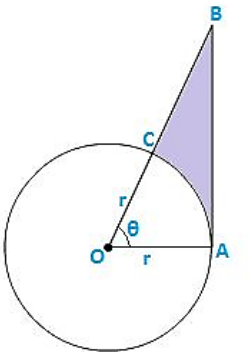The given figure shows a sector of a circle with centre 'O' subtending an angle θ°. Prove that:

1. Perimeter of shaded region is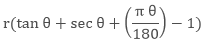2. Area of the shaded region is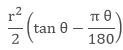Solution:

Given Data: Angle subtended at the centre of the circle = θ°

Angle OAB = 90° [at point of contact, tangent is perpendicular to radius]

OAB is a right angle triangle

cos θ = adjside/hypotenuse = r/OB = OB = r sec θ

sec θ = opposite/adjside = AB/r = AB = r tan θ

Perimeter of the shaded region = AB + BC + CA (arc)

=  r tan? + (OB - OC) + θ/360 ∗ 2πr cm

= r(tan θ + sec θ + π θ/180 − 1)

Area of the shaded region = (Area of triangle AOB) - (Area of sector)

(1/2 ∗ OA ∗ AB) − θ/360 ∗ πr2

On solving the above equation we get, r2/2[tan θ − πθ/180]

Question: 20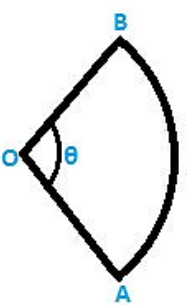The diagram shows a sector of circle of radius 'r' cm subtends an angle θ. The area of sector is A cm2 and perimeter of sector is 50 cm. Prove that θ = 360/π (25/r - 1) and A = 25 r - r2

Solution:

Given Data: Radius of circle = 'r' cm

Angle subtended at centre of the circle = θ

Perimeter = OA + OB + (AB arc)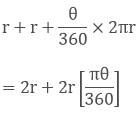As given in the question, perimeter = 50Area of the sector = θ/360 ∗ πr2

On solving the above equation, we have A = 25r - r2

Hence, proved.
```### Course Features

• 728 Video Lectures
• Revision Notes
• Previous Year Papers
• Mind Map
• Study Planner
• NCERT Solutions
• Discussion Forum
• Test paper with Video Solution